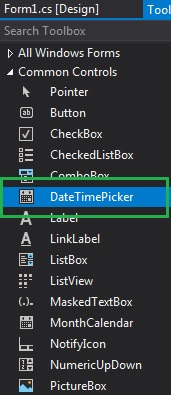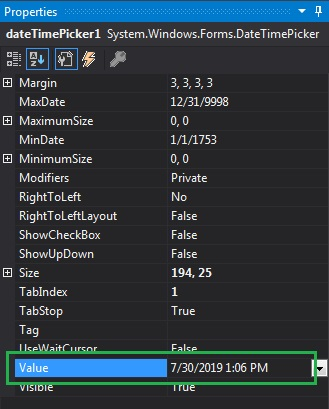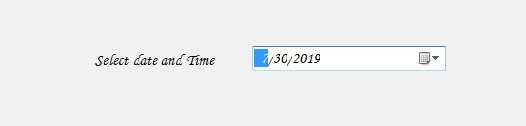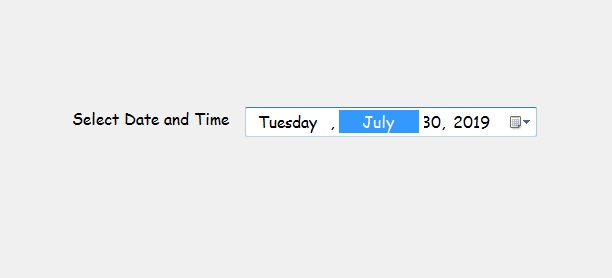# How to display Current Date/Time in the DateTimePicker in C#?

• Difficulty Level : Expert
• Last Updated : 02 Aug, 2019

In Windows Forms, the DateTimePicker control is used to select and display date/time with a specific format in your form. In DateTimePicker control, you can set the current Date/Time using the Value Property. or in other words, we can say that Value property is used to assign a Date/Time value to the DateTimePicker control. The default value of this property is the current date/time. You can set this property in two different ways:

1. Design-Time: It is the easiest way to set the value for the DateTimePicker as shown in the following steps:

• Step 1: Create a windows form as shown in the below image:
Visual Studio -> File -> New -> Project -> WindowsFormApp• Step 2: Next, drag and drop the DateTimePicker control from the toolbox to the form as shown in the below image:• Step 3: After drag and drop you will go to the properties of the DateTimePicker and set the value for the DateTimePicker as shown in the below image:Output:2. Run-Time: It is a little bit trickier than the above method. In this method, you can set a date and time value for the DateTimePicker control programmatically with the help of given syntax:

`public DateTime Value { get; set; }`

It will throw an ArgumentOutOfRangeException if the value of this property is less than MinDate or more than MaxDate. The following steps show how to set the value for the DateTimePicker dynamically:

• Step 1: Create a DateTimePicker using the DateTimePicker() constructor is provided by the DateTimePicker class.
```// Creating a DateTimePicker
DateTimePicker dt = new DateTimePicker();
```
• Step 2: After creating DateTimePicker, set the Value property of the DateTimePicker provided by the DateTimePicker class.
```// Setting the value
dt.Value = DateTime.Today;
```
• Step 3: And last add this DateTimePicker control to the form using the following statement:
```// Adding this control to the form
```

Example:

 `using` `System;``using` `System.Collections.Generic;``using` `System.ComponentModel;``using` `System.Data;``using` `System.Drawing;``using` `System.Linq;``using` `System.Text;``using` `System.Threading.Tasks;``using` `System.Windows.Forms;`` ` `namespace` `WindowsFormsApp48 {`` ` `public` `partial` `class` `Form1 : Form {`` ` `    ``public` `Form1()``    ``{``        ``InitializeComponent();``    ``}`` ` `    ``private` `void` `Form1_Load(``object` `sender, EventArgs e)``    ``{``        ``// Creating and setting the``        ``// properties of the Label``        ``Label lab = ``new` `Label();``        ``lab.Location = ``new` `Point(183, 162);``        ``lab.Size = ``new` `Size(172, 20);``        ``lab.Text = ``"Select Date and Time"``;``        ``lab.Font = ``new` `Font(``"Comic Sans MS"``, 12);`` ` `        ``// Adding this control to the form``        ``this``.Controls.Add(lab);`` ` `        ``// Creating and setting the ``        ``// properties of the DateTimePicker``        ``DateTimePicker dt = ``new` `DateTimePicker();``        ``dt.Location = ``new` `Point(360, 162);``        ``dt.Size = ``new` `Size(292, 26);``        ``dt.MaxDate = ``new` `DateTime(2500, 12, 20);``        ``dt.MinDate = ``new` `DateTime(1753, 1, 1);``        ``dt.Format = DateTimePickerFormat.Long;``        ``dt.Name = ``"MyPicker"``;``        ``dt.Font = ``new` `Font(``"Comic Sans MS"``, 12);``        ``dt.Visible = ``true``;``        ``dt.Value = DateTime.Today;`` ` `        ``// Adding this control ``        ``// to the form``        ``this``.Controls.Add(dt);``    ``}``}``}`

Output:My Personal Notes arrow_drop_up